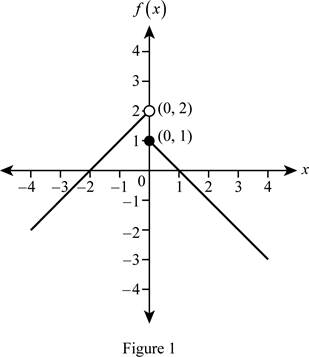# The values of f ( − 3 ) , f ( 0 ) , f ( 2 ) and sketch the graph of piecewise function.### Single Variable Calculus: Concepts...

4th Edition
James Stewart
Publisher: Cengage Learning
ISBN: 9781337687805### Single Variable Calculus: Concepts...

4th Edition
James Stewart
Publisher: Cengage Learning
ISBN: 9781337687805

#### Solutions

Chapter 1.1, Problem 43E
To determine

## To find: The values of f(−3),f(0),f(2) and sketch the graph of piecewise function.

Expert Solution

The value of f(3)=1 .

The value of f(0)=1 .

The value of f(2)=1 .

### Explanation of Solution

Given:

The function f(x)={x+2if x<01xif x0 .

Calculation:

Obtain the values of f(3),f(0), and f(2) as follows:

Since the value of x (=3) is less than 0, substitute −3 for x in the function f(x) = x + 2.

f(3)=3+2=1

The value of f(3)=1 .

Since the value of x is equal to 0, substitute 0 for x in the function f(x) = 1 − x.

f(0)=10=1

The value of f(0)=1 .

Since the value of x (=2) is greater than 0, substitute 2 for x in the function f(x) = 1 − x.

f(2)=12=1

The value of f(2)=1 .

If x<0 , then f(x)=x+2 is a line with slope 1 and y-intercept 2. And it lies to the left of the vertical line x = 0.

If x0 , then f(x)=1x is a line 1 – x with slope −1 and y-intercept 1. And it lies to the right of the vertical line x = 0.

Therefore, the graph of the function f(x) is shown below in Figure 1.In Figure 1, an open dot indicates that the point (0, 2) is excluded.

### Have a homework question?

Subscribe to bartleby learn! Ask subject matter experts 30 homework questions each month. Plus, you’ll have access to millions of step-by-step textbook answers!